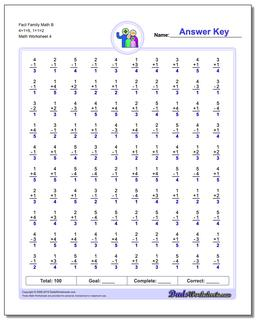PLEASE GO BACK AND USE THE BIG BLUE 'PRINT' BUTTON ON THE PAGE TO PRINT THE WORKSHEET CORRECTLY!Sorry for the trouble! The browser won't print the embedded worksheet PDF directly using the normal 'Print' command in the file menu, so you need to click the big 'Print' button to send just the worksheet and not the surrounding page to the printer.

# Math Worksheets: Fact Family Math: Fact Family Math: Fact Family Math B 4+1=5, 1+1=2 (Fourth Worksheet)## Fact Family Math B 4+1=5, 1+1=2 (Fourth Worksheet)

Property Value
DescriptionFact Family Math B 4+1=5, 1+1=2: These math worksheets have 100 addition and subtraction fact family problems and make for a challenging two minute test. (Fourth Worksheet)
Resource TypeWorksheet